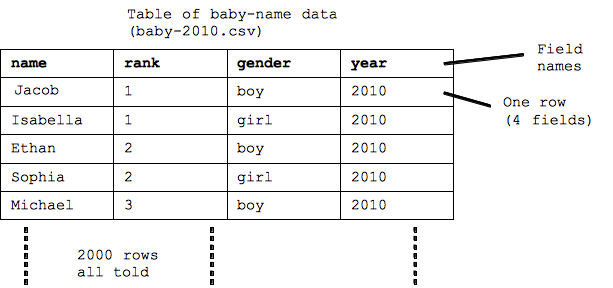# Table Data

• New form of data -- "table"
• Code that works on tables
--Similar to image-code ... basic patterns
• Tables are very common way to organize data on the computer

As another example of how data is stored and manipulated in the computer, we'll look at "table data" -- a common a way to organize strings, numbers, dates in rectangular table structure. In particular, we'll start with data from the social security administration baby name site.

## Social Security Baby Name Example

• Names for babies born each year
• Top 1000 boy and girl names, 2000 names total
• Organized as a "table"
Fields: name, rank, gender, year (columns)
Rows: one row holds the data for one name• The table is made of 2000 rows, each row represents the data of one name
• Each row is divided into 4 fields
• Each of the 4 fields has its own name. The field names are: name, rank, gender, year

## Tables Are Very Common

• Rectangular table format is very common
• "Databases" -- extension of this basic table idea
• Number of fields is small (categories)
• Number of rows can be millions or billions
• e.g. email inbox: one row = one message, fields: date, subject, from, ...
• e.g. craigslist: one row = one thing for sale: description, price, seller, date, ...

Much of the information stored on computers uses this table structure. One "thing" we want to store -- a baby name, someone's contact info, a craigslist advertisement -- is one row. The number of fields that make up a row is fairly small -- essentially the fixed categories of information we think up for that sort of thing. For example one craigslist advertisement (stored in one row) has a few fields: a short description, a long description, a price, a seller, ... plus a few more fields.

The number of fields is small, but the number of rows can be quite large -- thousands or millions. When someone talks about a "database" on the computer, that builds on this basic idea of a table. Also storing data in a spreadsheet typically uses exactly this table structure.

## Table Code

We'll start with some code -- SimpleTable -- which will serve as a foundation for you to write table code. Run the code to see what it does.

• Baby data stored in "baby-2010.csv"
--".csv" stands for "comma separated values" and it is a simple and widely used standard format to store a table as text in a file.
• Recall we had: `for (pixel: image) { code`
• For tables: `for (row: table) { code`
• print(row) prints out the fields of a row on one line

 table = new SimpleTable("baby-2010.csv"); for (row: table) { print(row); }

## Table Query Logic

• Select the rows we want (if-statement)
• Database terminology -- a "query" on the database
• e.g. select rows where the rank is 6
`if (row.getField("rank") == 6) { ...`

 table = new SimpleTable("baby-2010.csv"); for (row: table) { if (row.getField("rank") == 6) { print(row); } }

The above code loops over all the rows, and the if-statement prints just the rows where the test is true -- here testing if the rank field is equal to 6, but really the if-statement could test anything about the row.

• `row.getField("field-name")` -- pick field out of row
• Field names for the baby table: name, rank, gender, year
• `==` are two values equal? (two equal signs)
• Warning: single equal sign `=` does variable assignment, not comparison. Use `==` inside if-test. (warning)
• Other comparisons: < > <= >=
• e.g. select row where the name is "Alice":
`if (row.getField("name") == "Alice") { ...`

 table = new SimpleTable("baby-2010.csv"); for (row: table) { if (row.getField("name") == "Alice") { print(row); } }

The row object has a `row.getField("field-name")` function which returns the data for one field out of the row. Each field has a name -- one of "name" "rank" "gender" "year" in this case -- and the string name of the field is passed in to getField() to indicate which field we want, e.g. `row.getField("rank")` to retrieve the rank out of that row.

You can test if two values are equal in JavaScript with two equal signs joined like this: `==`. Using ==, the code to test if the name field is "Alice" is `row.getField("name") == "Alice"`

Note that a single equal sign `=` does variable assignment and not comparison. It's a common mistake to type in one equal sign for a test, when you mean two equal signs. For this class, the Run button will detect an accidental use of a single = in an if-test and give an error message. The regular less-than/greater-than type tests: < > <= >= work as have seen before.

## Table Query Examples

 table = new SimpleTable("baby-2010.csv"); for (row: table) { if (row.getField("name") == "Alice") { print(row); } }

Write in code above to solve these problems:

• Baby table fields: name, rank, gender, year
• name field is "Alice", "Robert", "Bob", "Abby", "Abbey" (try each in turn, yes nobody names their child "Bob" .. apparently always using Robert or Bobby)
• rank field is 1
• rank field is < 10
• rank field is <= 10
• rank field is > 990
• gender field is "girl"
• What is going on for all these: the loop goes through all 2000 rows and evaluates the if-test for each, printing that row only if the test is true.

Solution code: